# Barrier

Lebesgue barrier, in potential theory

A function the existence of which is a necessary and sufficient condition for the regularity of a boundary point with respect to the behaviour of a generalized solution of the Dirichlet problem at that point (cf. Perron method; Regular boundary point).

Letbe a domain in a Euclidean space,, and letbe a point on its boundary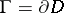. A barrier for the pointis any function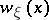, continuous in the intersection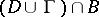of the closed domain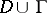with some ball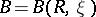with centre at, which is superharmonic inand positive in, except at, at which it vanishes. For instance, ifandis any boundary point for which there exists a closed ball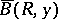inwhich meetsonly in, one can take as a barrier the harmonic function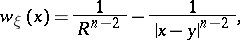where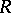is the radius ofandis its centre.

A barrier in the theory of functions of (several) complex variables is a function the existence of which for all boundary points of the domainimplies thatis a domain of holomorphy. Letbe a domain in the complex space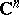,, and letbe a point of the boundary. Any analytic function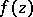inwith a singular point atwill then be a barrier at. Thus, the functionis a barrier for the boundary pointof any plane domain. There also exists a barrier at any point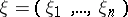of the boundary of the balle.g. the function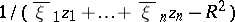.

A barrier exists at a boundary pointof a domainif there is an analytic function defined inthat is unbounded at, i.e. is such that for some sequence of points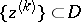which converges toone has: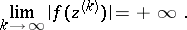The converse is true for domains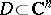in the following strong form: For any set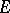of boundary points of a domainat which a barrier exists, one can find a function holomorphic inwhich is unbounded at all points of. Ifis everywhere dense in the boundary of, thenis a domain of holomorphy.

How to Cite This Entry:
Barrier. Encyclopedia of Mathematics. URL: http://encyclopediaofmath.org/index.php?title=Barrier&oldid=28154
This article was adapted from an original article by E.D. SolomentsevM. Shirinbekov (originator), which appeared in Encyclopedia of Mathematics - ISBN 1402006098. See original article